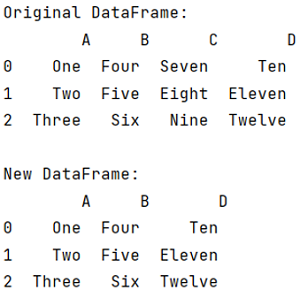# How to extract specific columns to new DataFrame?

Given a Pandas DataFrame, we have to extract specific columns to new DataFrame. By Pranit Sharma Last updated : September 20, 2023

Columns are the different fields that contains their particular values when we create a DataFrame. We can perform certain operations on both rows & column values. In pandas, we can make a copy of some specific columns of an old DataFrame. This is an easy task in pandas. Let us understand with the help of an example.

## Problem statement

Given a Pandas DataFrame, we have to extract specific columns to new DataFrame.

## Extracting specific columns to new DataFrame

For this purpose, we will pass the list of columns (whose value we have to extract) as the index of the DataFrame and assign the result to the another/new DataFrame. Finally, print the newly created DataFrame.

Note

To work with pandas, we need to import pandas package first, below is the syntax:

```import pandas as pd
```

Let us understand with the help of an example.

## Python program to extract specific columns to new DataFrame

```# Importing Pandas package
import pandas as pd

# Create a dictionary
d = {
'A':['One','Two','Three'],
'B':['Four','Five','Six'],
'C':['Seven','Eight','Nine'],
'D':['Ten','Eleven','Twelve']
}

# Create DataFrame
df1 = pd.DataFrame(d)

# Display DataFrame
print("Original DataFrame:\n",df1,"\n")

# Now converting another DataFrame consisting of
# some specific columns of DataFrame 1
df2 = df1[['A','B','D']]

# Display New DataFrame
print("New DataFrame:\n",df2)
```

### Output

The output of the above program is: